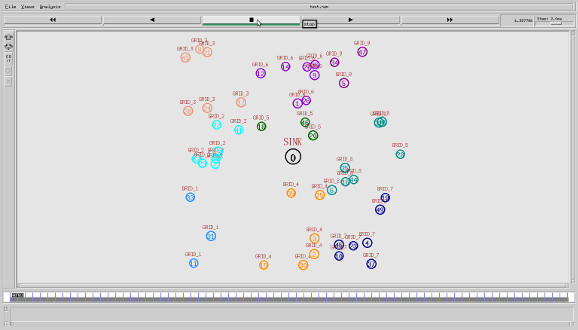• #5, First Floor, 4th Street Dr. Subbarayan Nagar Kodambakkam, Chennai-600 024 Landmark : Samiyar Madam
• pro@slogix.in
• +91- 81240 01111

### How to form grid based clustering in the network using NS2?

#### Description

In large network cluster formation is done in grid format with availability location information of the nodes. In distributed case, each node executes the algorithm given below by applying its own position to find the grid it belongs to. In centralized case, sink node applies the algorithm and informs the grid id to each node. The grid formation is based on communication range, network width and network height.

###### Sample Code

for {set i 1} {\$i < \$val(nn) } { incr i } {

set grid 1

for {set gx 1} {\$gx <= \$g } { incr gx } {

if { \$xx(\$i) <= [expr \$gwidth * \$gx] } {

set xp [expr \$gwidth * \$gx]

set gx [expr round(\$g) + 1]

for {set gy 1} {\$gy <= \$g } { incr gy } {

set yp [expr \$gheight * \$gy]

if { \$yy(\$i) <= [expr \$gheight * \$gy] } {

set gridid(\$i) \$grid

break;

} else {

set grid [expr \$grid + 1]

}

}

} else {

set grid [expr [expr \$gx * \$g] + 1]

}

}

}

###### Screenshots

Here, total grids are 9.